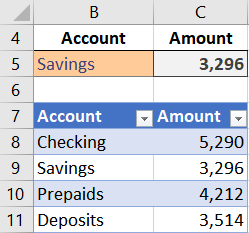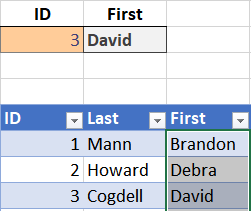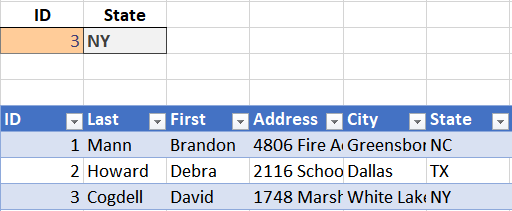## VLOOKUP Hack #4: Column Labels

This is the 4th post in the VLOOKUP Hacks series. The first three posts have explored the 4th argument. Now, we are going to explore a hack for the 3rd argument. In this post, we’ll hack the 3rd argument so that it references column labels instead of the column position. Check it.

Note: depending on your version of Excel, you may have XLOOKUP as an option … more info here: https://www.excel-university.com/xlookup/

## Objective

Here’s the deal. The 3rd argument of the VLOOKUP function is officially known as col_index_num. This represents the position of the value you want returned. For example, if you want to return the amount from the 2nd position, or column, within the lookup range, you would enter 2 for the argument. Consider the screenshot below.To return the amount from the 2nd column in Table1, we could use the following formula written into C5:

`=VLOOKUP(B5, Table1, 2, 0)`

But, what if we wanted to communicate with Excel using the column label (Amount) instead of an integer value (2). For example, we wanted to do something like this:

`=VLOOKUP(B5, Table1, "Amount", 0)`

If we enter such a formula we get an error. So, apparently Excel does not allow us to reference the column using the column label. Or…does it?

That question brings us to our next hack. I’ve included a video demonstration as well as a written narrative for reference.

## Hack

Essentially, we would like Excel to translate the column label (Amount) into the corresponding integer (2). Here’s how.

Hack: use MATCH instead of an integer for the 3rd argument.

The MATCH function returns the relative position of a list item. So, we will ask the MATCH function to find the label (Amount) within the table’s header row, and return the position number. Then, VLOOKUP will use that position number.

For example, the following formula would return 2 since Amount is the second column label within the table:

`=MATCH("Amount", Table1[#Headers], 0)`

But, since “Amount” is already entered into cell C4, we can simple reference C4 instead, as follows:

`=MATCH(C4, Table1[#Headers], 0)`

This MATCH function would return 2 since the Amount label is in the 2nd table column. So, replacing the 2 in our original formula with the MATCH function would look like this:

`=VLOOKUP(B5, Table1, MATCH(C4,Table1[#Headers],0), 0)`

This technique allows us to reference the column labels instead of the position number. But, Jeff, hang on. That seems WAY more complicated than just using an integer. Why would we want to go through such trouble? Well, let’s check out a few fun applications of this technique.

## Applications

Application 1: Column order changes

If the column order changes, a traditional VLOOKUP function (written with an integer 3rd argument) will break. Why? Because Excel doesn’t update the integer value accordingly. Using MATCH instead prevents this error, making your workbook more reliable and efficient.

For example, First name is originally in the 2nd column. But, then we move it to the 3rd column, as illustrated below.Using the MATCH function as the 3rd argument allows the VLOOKUP to continue to retrieve the desired First name, even if the column order changes, as shown below.Note: the lookup column must continue to be the first column within the lookup range.

Application 2: Insert new columns

If a new worksheet column is inserted between the lookup column and the return column, a traditional VLOOKUP function will break because Excel won’t update the integer value accordingly.

But, using MATCH instead of an integer enables the VLOOKUP function to automatically adapt. This reduces the likelihood of an error and improves efficiency since you won’t need to rewrite the formula after inserting a new column.

For example, we return the State from the 6th table column, as shown below.Later, we insert a new column Address2. Our hacked VLOOKUP function continues to return the State code (now in the 7th table column) without modification, as shown below.Application 3: Two-dimensional lookups

When we think about traditional VLOOKUP functions, we think of them as searching for a matching value down, vertically. But, with MATCH, we can perform two-dimensional lookups, where VLOOKUP searches down through the rows and MATCH searches across the columns.

This opens up some interesting possibilities.  For example, let’s say we have some inventory items that we sell for different amounts, depending on the discount level of the customer. We could create a master table of items, and use one column for each pricing level or tier. Then, we could define the tier in an input cell (C7), and have Excel search down the list for the matching item, and then right to find the price based on the specified tier.And these are just a few ways to apply the MATCH hack to VLOOKUP! If you have any other VLOOKUP hacks, please share by posting a comment below…thanks!

To check out the formulas for all of the examples above, just download the sample Excel file below.

Posted in ,### Jeff Lenning

I love sharing the things I've learned about Excel, and I built Excel University to help me do that. My motto is: Learn Excel. Work Faster.

### Want to learn Excel?

Our Campus Pass includes access to our entire Undergrad and Masters catalog. Gamification ensures it is the most fun you can have learning Excel :)

1.Ian on November 9, 2017 at 1:20 pm

Good one – thanks.

•Jeff Lenning on November 9, 2017 at 1:31 pm

Welcome 🙂

2.Pam on November 9, 2017 at 2:40 pm

I love this series. Just wish my company would allow me to watch the videos – they get blocked! Fortunately, the written narrative is complete and you provide the sample files, which we are allowed to download. Thank you!!

•Jeff Lenning on November 9, 2017 at 2:52 pm

Thanks Pam … and I’ll leave the videos on YouTube going forward in case you want to check them out later 🙂
Thanks,
Jeff

3.Jeremey on July 31, 2020 at 10:48 am

=IFERROR(VLOOKUP(C2,MATCH(New Worksheet!\$K\$1,New Worksheet!\$1:\$1,0),0),0)

I am trying to add the IFERROR function on top this hack, but whatever I have the return for the IFERROR argument overrides the match hack. Please help.

!
!